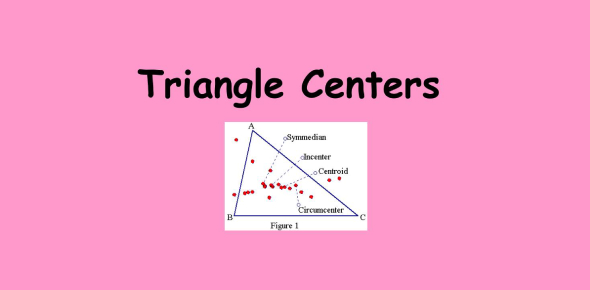# Trivia Quiz On Triangle Centers! Math Trivia

Approved & Edited by ProProfs Editorial Team
At ProProfs Quizzes, our dedicated in-house team of experts takes pride in their work. With a sharp eye for detail, they meticulously review each quiz. This ensures that every quiz, taken by over 100 million users, meets our standards of accuracy, clarity, and engagement.
| Written by Karla Lopez
K
Karla Lopez
Community Contributor
Quizzes Created: 1 | Total Attempts: 442
Questions: 10 | Attempts: 442Settings.

• 1.

### What is the name of the segment that joins the midpoints of the sides of a triangle?

• A.

Median

• B.

Midsegment

• C.

Vertex

• D.

Centroid

B. Midsegment
Explanation
The correct answer is "Midsegment". A midsegment is a line segment that connects the midpoints of two sides of a triangle. It is also known as a midline. The midsegment is parallel to the third side of the triangle and is half the length of that side. It divides the triangle into two smaller triangles of equal area.

Rate this question:

• 2.

### What is the name of the segment that joins a vertex to the midpoint of the opposite side in a triangle?

• A.

Midsegment

• B.

Median

• C.

Vertex

• D.

Centroid

B. Median
Explanation
A median is a segment that joins a vertex of a triangle to the midpoint of the opposite side. It divides the triangle into two equal areas and is also the segment where the three medians of a triangle intersect.

Rate this question:

• 3.

### What is it called when three or more lines intersect at the same point?

• A.

Concurrent

• B.

Equilateral

• C.

Equiangular

• D.

Conspicuous

A. Concurrent
Explanation
When three or more lines intersect at the same point, it is called "concurrent." This means that all the lines meet at a common point, creating an intersection. The term "concurrent" is used to describe this specific geometric arrangement of intersecting lines.

Rate this question:

• 4.

### What is the point of concurrency of the altitudes?

• A.

Incenter

• B.

Centroid

• C.

Circumcenter

• D.

Orthocenter

D. Orthocenter
Explanation
The point of concurrency of the altitudes in a triangle is called the orthocenter. The altitudes of a triangle are the perpendicular lines drawn from each vertex to the opposite side. The orthocenter is the point where these three altitudes intersect. It is an important point in a triangle as it has special properties and is used in various geometric constructions and calculations.

Rate this question:

• 5.

### What is the name of the segment drawn from the vertex of a triangle, perpendicular to the opposite side?

• A.

Median

• B.

Midsegment

• C.

Altitude

• D.

Midpoint

C. Altitude
Explanation
The segment drawn from the vertex of a triangle, perpendicular to the opposite side, is called an altitude.

Rate this question:

• 6.

### What is the point of concurrency of the medians?

• A.

Incenter

• B.

Orthocenter

• C.

Centroid

• D.

Circumcenter

C. Centroid
Explanation
The point of concurrency of the medians is the centroid. The medians of a triangle are the line segments that connect each vertex to the midpoint of the opposite side. The centroid is the point where all three medians intersect. It is also known as the center of gravity of the triangle. The centroid divides each median into two segments, with the ratio of 2:1.

Rate this question:

• 7.

### A midsegment's length is _____ that of its parallel side.

• A.

Two-thirds

• B.

Two

• C.

One-half

• D.

One-third

C. One-half
Explanation
A midsegment is a line segment that connects the midpoints of two sides of a triangle. It is parallel to the third side of the triangle. Since the midsegment connects the midpoints, it divides the third side into two equal parts. Therefore, the length of the midsegment is half the length of the parallel side.

Rate this question:

• 8.

### This is which formula?

• A.

Midpoint

• B.

Slope

• C.

Distance

• D.

C. Distance
Explanation
The formula mentioned in the question is used to calculate the distance between two points. It is commonly known as the distance formula in mathematics. This formula is derived from the Pythagorean theorem and is used to find the straight-line distance between two points in a coordinate plane. It involves finding the difference between the x-coordinates and the y-coordinates of the two points, squaring those differences, summing them, and then taking the square root of the sum.

Rate this question:

• 9.

### Which formula is this?

• A.

Slope

• B.

Distance

• C.

• D.

Midpoint

D. Midpoint
Explanation
This formula is the midpoint formula, which is used to find the coordinates of the midpoint between two given points on a line. It is commonly used in geometry and coordinate geometry to determine the center point or middle point between two endpoints.

Rate this question:

• 10.

### For what do you use this formula?

• A.

Finding midpoints

• B.

Calculating distance

• C.

Finding slope

• D.

Measuring angleBack to top